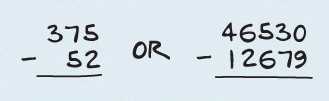Numbers: An introduction to subtraction

This free course is available to start right now. Review the full course description and key learning outcomes and create an account and enrol if you want a free statement of participation.

Free course

# 4 Subtracting on paper

If the numbers you want to subtract are too large for you to do the calculation in your head, you can use a calculator. Alternatively, you can do the calculation on paper.

Write your starting number at the top with the number you want to subtract from it underneath. Because the order in which you subtract one number from another matters, it is important to put the correct numbers on top and bottom. Then draw a line underneath.

Write the numbers so the digits form columns and they are lined up on the right-hand side, as in the examples shown below. In the calculation below left, for instance, the digit 5 in the top number is above the digit 2 in the bottom number.The far right column represents the ones (units), the next column to the left represents the tens, the next column to the left the hundreds, the next column to the left the thousands, and so on.

To do the subtraction, you take each column in turn, starting from the right and moving left. You take the bottom digit from the top digit and write the result underneath the line, lined up with the digits above.

If the top number in each column is bigger than the bottom number, like in the calculation above left, this is straightforward. There’s an example of this kind of straightforward subtraction in the next section.

But what do you do if the number at the top of the column is smaller than the number at the bottom, like in some of the columns in the calculation on the right above? This requires an extra step. There’s an example showing what you need to do in this case later, in Section 6 Subtracting when you have to borrow.

Sub_1

### Take your learning further

Making the decision to study can be a big step, which is why you'll want a trusted University. The Open University has 50 years’ experience delivering flexible learning and 170,000 students are studying with us right now. Take a look at all Open University courses.

If you are new to university level study, find out more about the types of qualifications we offer, including our entry level Access courses and Certificates.

Not ready for University study then browse over 900 free courses on OpenLearn and sign up to our newsletter to hear about new free courses as they are released.

Every year, thousands of students decide to study with The Open University. With over 120 qualifications, we’ve got the right course for you.

Request an Open University prospectus